# Create Expression Plot

Create Expression Plot generates visualizations of gene expressions for a small number of genes. It uses expressions from an input Expression Matrix (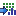) and groupings provided by Cell Clusters (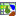) or Cell Annotations (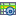).

The tool can output:

• A Heat Map (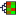) with one row per gene and one column per cell.
• A Dot Plot (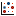) with one row per gene and one column per grouping of cells.
• A Violin Plot (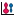) with one violin distribution curve per combination of gene and group.

It is often most natural to run the tool from a Dimensionality Reduction Plot, by right-clicking on the plot. However, it can also be found in the Toolbox here:

Expression Analysis (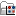) | Create Expression Plot (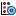)

The first set of options control how cells are grouped. The groupings are shown at the top of the Heat Map, form the columns of the Dot Plot and define groups in the Violin Plot. These options are:

• Clusters and Cell annotations. At least one of these must be supplied. Clusters accepts Cell Clusters () and Cell annotations accepts Cell Annotations ().
• Group by. One or more columns from the supplied Cell Clusters or Cell Annotations. Columns that only contain non-integer numerical data are not supported. If Cell Clusters contained a column Cell type' with values T cell', B cell' and Platelet', and Cell Annotations contained a column Status' with values Case' and Control', then selecting Group by = Cell type, Status would give groups T cell - Case', T cell - Control', B cell - Case', B cell - Control', Platelet - Case', and Platelet - Control'.
• Select groups (Optional). This can be supplied to reduce the number of groups of cells in the plot to only those of interest, or to control the order in which the groups are shown. For example, if the aim of the plot is to show how expression changes in T cells as a function of case / control, the T cell - Case' and T cell - Control' groups can be selected. If left empty, all groups will be displayed.

The genes in the output Heat Map or Dot Plot are clustered such that genes with similar expression patterns are found on adjacent rows. The clustering has a tree structure that is generated by

1. Letting each feature or sample be a cluster.
2. Calculating pairwise distances between all clusters.
3. Joining the two closest clusters into one new cluster.
4. Iterating 2-3 until there is only one cluster left (which contains all the genes).

In the Heat Map, the clustering is drawn as a tree where distances between clusters are reflected by the lengths of the branches in the tree.

The above algorithm requires a distance measure and a linkage' that describes how to apply the distance measure to clusters.

There are three kinds of Distance measures:

• Euclidean distance. The ordinary distance between two points - the length of the segment connecting them. If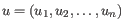and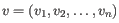, then the Euclidean distance between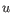and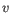is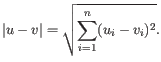• 1 - Pearson correlation. The Pearson correlation coefficient between two elements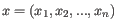and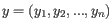is defined as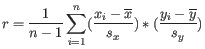where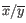is the average of values in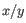and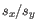is the sample standard deviation of these values. It takes a value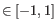. Highly correlated elements have a high absolute value of the Pearson correlation, and elements whose values are un-informative about each other have Pearson correlation 0. Using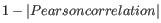as distance measure means that elements that are highly correlated will have a short distance between them, and elements that have low correlation will be more distant from each other.
• Manhattan distance. The Manhattan distance between two points is the distance measured along axes at right angles. Ifand, then the Manhattan distance betweenandis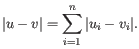• Single linkage. The distance between two clusters is computed as the distance between the two closest elements in the two clusters.
• Average linkage. The distance between two clusters is computed as the average distance between objects from the first cluster and objects from the second cluster. The averaging is performed over all pairs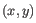, where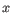is an object from the first cluster and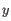is an object from the second cluster.
• Complete linkage. The distance between two clusters is computed as the maximal object-to-object distance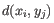, where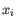comes from the first cluster, and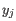comes from the second cluster. In other words, the distance between two clusters is computed as the distance between the two farthest objects in the two clusters.

There are usually too many cells for all of them to be viewed in a Heat Map on a standard computer display. Max cells in heat map constructs the Heat Map by sampling the given number of cells from the full Expression Matrix. This option has no effect on the Dot Plot. Sampling works by sampling a fixed percentage of the cells in each grouping. For example, if there are 10 000 cells in the input, and `Max cells in heat map = 1 000', then sampling will aim to recover 1 000 / 10 000 = 10% of the cells for each grouping. In this example, a group with <5 cells would be omitted, because 10% of <5 would be rounded down to 0.

There are also usually too many features to allow for a meaningful visualization of all genes. Therefore several options can be used to select the most informative genes to visualize:

• Keep fixed number of features
• Fixed number of features This option is only available when data have been normalized by Normalize Single Cell Data. The given number of highly variable genes (HVGs) are selected according to the variance of their normalized values, from highest variance to lowest variance.
• Filter by statistics Keeps features that are differentially expressed according to the specified cut-offs. All the cut-offs must be satisfied in at least one of the input Statistical Comparison Tables.
• Statistical comparison One or more Statistical Comparison Table, such as are produced by Differential Expression for Single Cell.
• Minimum absolute fold change Only features with a higher absolute fold change are kept.
• Threshold Only features with a lower p-value are kept. It is possible to select which type of p-value to use.
• Specify features Keeps a set of features, as specified by either a feature track or by plain text.
• Feature track Any genes or transcripts defined in the feature track will be kept.
• Keep these features A plain text list of feature names. Any white-space characters, and ",", and ";" are accepted as separators.

Subsections